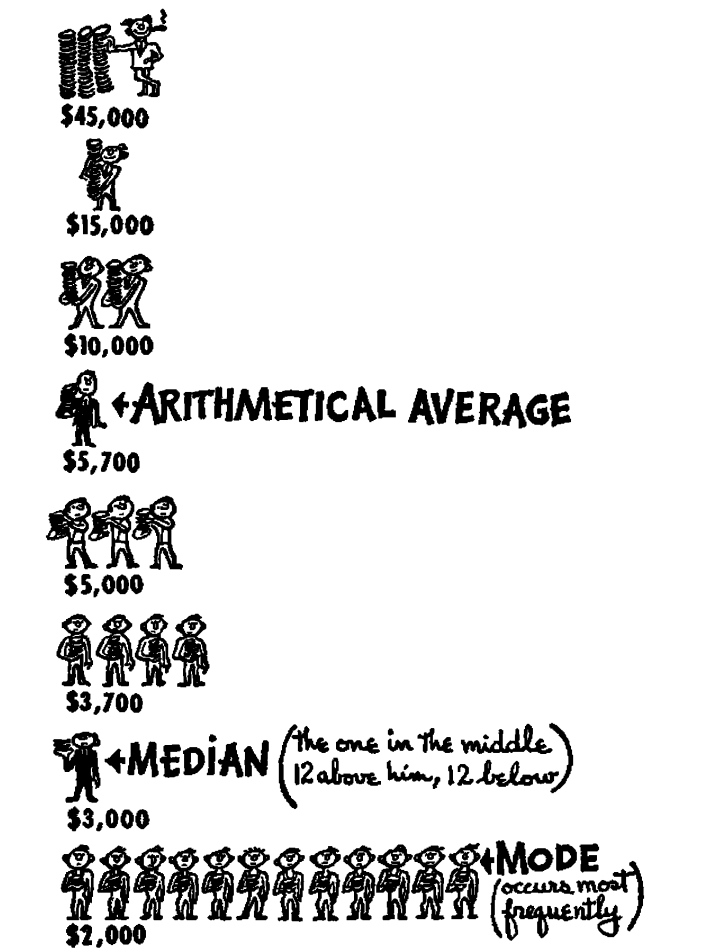## How to Lie with Statistics - The Well-Chosen Average explained with Python

written by Guido Percú on May 17, 2020

### The average dog owner spends \$500 a year on dog food. But the typical dog pamperer spends \$5,000 a year (all numbers invented). The reason the numbers are different is that the samples are different. We chop off the outliers in the second set, homing in on the kind of person we’re talking about.

#### Seth Godin - Average is not the same as typicalBe careful when you read news about "average wage". As we learn from the classic book How to Lie with Statistics, there are different types of averages and their different meanings can be used to mislead and deceive.

When you read an announcement describing the average payment of people working on a company (or an industry), that number may mean something different than you think. If that average is a median, then it means that half the employees earn less than it and half earn more than that. But if that number is a mean, you may be getting nothing more relevant than the average of the CEO with his underpaid employees.To better understand this concept, let's open our Python shell and experiment with the packages Numpy and Scipy. First, we import the necessary packages:

``````import numpy as np
from scipy import stats
``````

Then we define the incomes that we're studying as a numpy array. I'm going to use the same example from the book.

``````income = np.array([45000, 15000, 10000, 10000, 5700, 5000, 5000, 5000, 3700, 3700, 3700, 3700,
3000, 2000, 2000, 2000, 2000, 2000, 2000, 2000, 2000, 2000, 2000, 2000, 2000])
``````

Now we're going to get our different types of averages.

``````mean = np.mean(income)# arithmetic average
median = np.median(income) # half earns more, half earns less
mode = stats.mode(income) # most common income
``````

Printing those values (on a locale base currency format) will show you their differences.

``````import locale
locale.setlocale( locale.LC_ALL, '' )

print("Mean: {}".format(locale.currency( mean, grouping=True )))
print("Median: {}".format(locale.currency(median, grouping=True)))
print("Mode: {}".format(locale.currency(mode.mode, grouping=True)))
``````
``````Mean: R\$ 5.700,00
Median: R\$ 3.000,00
Mode: R\$ 2.000,00
``````